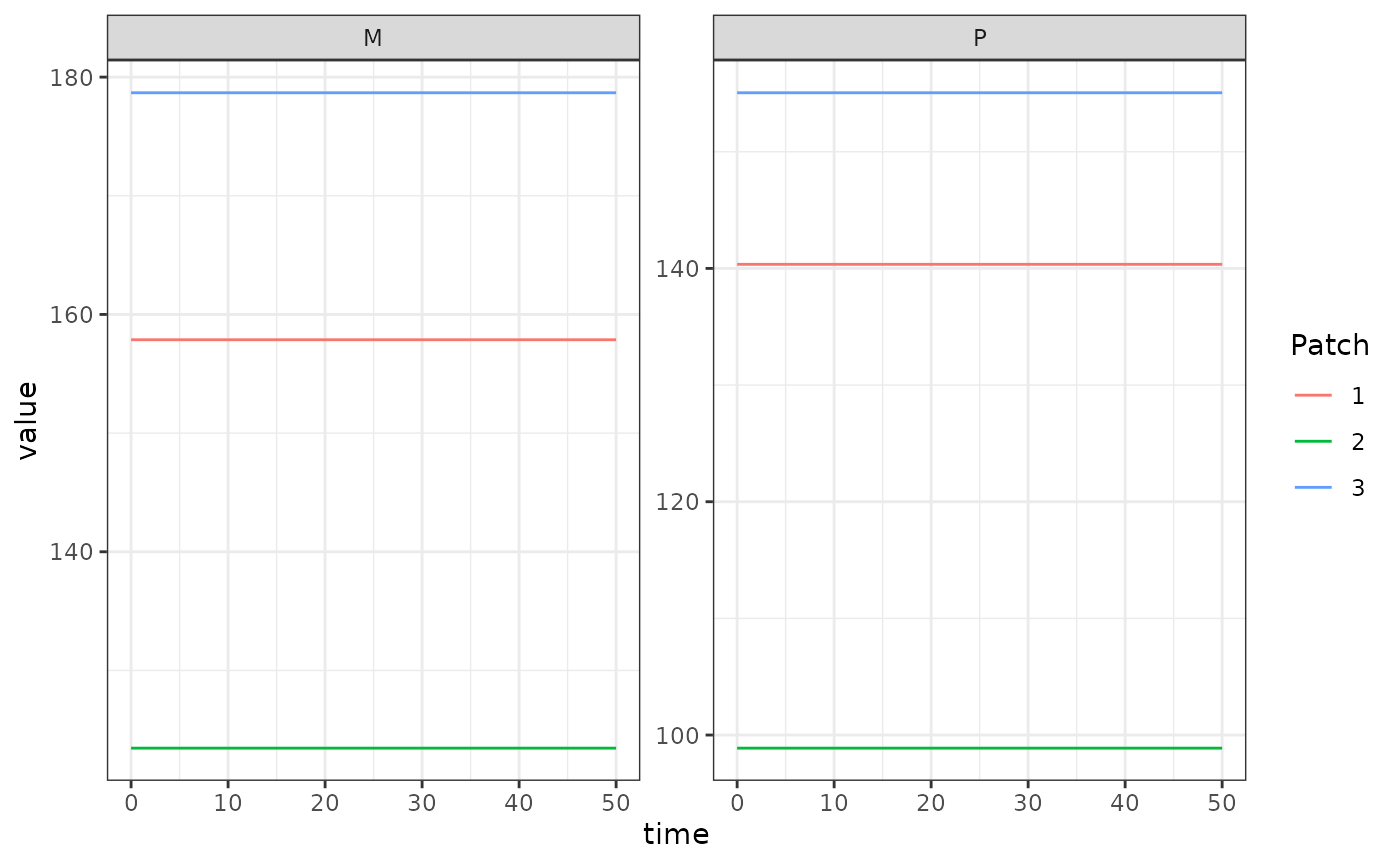## A forced model

library(exDE)
library(MASS)
library(expm)
library(deSolve)
library(data.table)
library(ggplot2)

This is the null model of aquatic mosquito dynamics; there are no endogenous dynamics and the model is simply specified by Lambda, the rate at which adult mosquitoes spontaneously emerge from aquatic habitats.

Below we show an example, taken from the package tests, of using the trace-based aquatic model to keep an adult mosquito model at equilibrium when using unequal numbers of aquatic habitats per patch.

### The Long Way

First, we set the parameter values.

nPatches <- 3
nHabitats <- 4
f <- 0.3
q <- 0.9
g <- 1/20
sigma <- 1/10
eip <- 11
nu <- 1/2
eggsPerBatch <- 30

calN <- matrix(0, nPatches, nHabitats)
calN[1,1] <- 1
calN[2,2] <- 1
calN[3,3] <- 1
calN[3,4] <- 1

calU <- matrix(0, nHabitats, nPatches)
calU[1,1] <- 1
calU[2,2] <- 1
calU[3:4,3] <- 0.5

calK <- matrix(0, nPatches, nPatches)
calK[1, 2:3] <- c(0.2, 0.8)
calK[2, c(1,3)] <- c(0.5, 0.5)
calK[3, 1:2] <- c(0.7, 0.3)
calK <- t(calK)

Omega <- make_Omega(g, sigma, calK, nPatches)
Upsilon <- expm::expm(-Omega * eip)

kappa <- c(0.1, 0.075, 0.025)
Lambda <- c(5, 10, 8)

Next, we calculate equilibrium values following the Ross-Macdonald vignette. We use $$\mathcal{N}$$ and $$\mathcal{U}$$ to describe how aquatic habitats are dispersed amongst patches, and how mosquitoes in each patch disperse eggs to each habitat, respectively. Please note because we have unequal numbers of aquatic habitats and patches, we use MASS::ginv to compute the generalized inverse of $$\mathcal{N}$$ to get $$\alpha$$ required at equilibrium.

# equilibrium solutions
Omega_inv <- solve(Omega)

M_eq <- as.vector(Omega_inv %*% Lambda)
P_eq <- as.vector(solve(diag(f, nPatches) + Omega) %*% diag(f, nPatches) %*% M_eq)

# the "Lambda" for the dLdt model
alpha <- as.vector(ginv(calN) %*% Lambda)

Now we set up the model. Please see the Ross-Macdonald vignette for details on the adult model. We use alpha as the Lambda parameter of our forced emergence model, in exDE::make_parameters_L_trace. Again, because we are not running the full transmission model, we must use exDE::MosquitoBehavior to pass the equilibrium values of those bionomic parameters to exDE::xDE_diffeqn_mosy.

params = make_parameters_xde()
params$nPatches = nPatches params$nHabitats = nHabitats
params$calU = calU params$calN = calN

# ODE
params$beta = beta params = make_Lpar_trace(pars = params, Lambda = alpha) params = make_MYZpar_basicM(pars = params, g = g, sigma = sigma, calK = calK, f = f, q = q, nu = nu, eggsPerBatch = eggsPerBatch) params = make_MYZinits_basicM(params, M0=M_eq, P0=P_eq) params = make_indices(params) y0 <- rep(0, params$max_ix)
y0[params$MYZpar$M_ix] <- M_eq
y0[params$MYZpar$P_ix] <- P_eq

out <- deSolve::ode(y = y0, times = 0:50, func = xDE_diffeqn_mosy, parms = params, method = 'lsoda')
out1 <- out
colnames(out)[params$MYZpar$M_ix+1] <- paste0('M_', 1:params$nPatches) colnames(out)[params$MYZpar$P_ix+1] <- paste0('P_', 1:params$nPatches)

out <- as.data.table(out)
out <- melt(out, id.vars = 'time')
out[, c("Component", "Patch") := tstrsplit(variable, '_', fixed = TRUE)]
out[, variable := NULL]

ggplot(data = out, mapping = aes(x = time, y = value, color = Patch)) +
geom_line() +
facet_wrap(. ~ Component, scales = 'free') +
theme_bw()### Using Setup

Lo = list(
Lambda = alpha
)
MYZo = list(
f = 0.3,
q = 0.9,
g = 1/20,
sigma = 1/10,
eip = 11,
nu = 1/2,
eggsPerBatch = 30,
M0=M_eq,
P0=P_eq
)
xde_setup_mosy("mosy1",
MYZname = "basicM", Lname = "trace",
nPatches = 3, membership = c(1,2,3,3),
MYZopts = MYZo, calK = calK, Lopts = Lo,
kappa = c(0.1, 0.075, 0.025))->mosy1
xde_solve(mosy1,Tmax=50,dt=1)$outputs$orbits\$deout -> out2
sum(out2-out1)
#>  0